# Texas Go Math Grade 8 Lesson 8.3 Answer Key Distance Between Two Points

Refer to our Texas Go Math Grade 8 Answer Key Pdf to score good marks in the exams. Test yourself by practicing the problems from Texas Go Math Grade 8 Lesson 8.3 Answer Key Distance Between Two Points.

## Texas Go Math Grade 8 Lesson 8.3 Answer Key Distance Between Two Points

Example 1

The figure shows a right triangle. Approximate the length of the hypotenuse to the nearest tenth without using a calculator.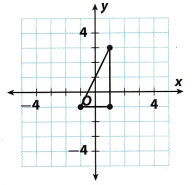STEP 1: Find the length of each leg.
The length of the vertical leg is 4 units.
The length of the horizontal leg is 2 units.

STEP 2: Let a = 4 and b = 2. Let c represent the length of the hypotenuse. Use the Pythagorean Theorem to find c.STEP 3: Approximate $$\sqrt {20}$$ by finding perfect squares close to 20.
$$\sqrt {20}$$ is between $$\sqrt {16}$$ and $$\sqrt {25}$$ or $$\sqrt {16}$$ < $$\sqrt {20}$$ < $$\sqrt {25}$$ .
Simplifying gives 4 < $$\sqrt {20}$$ < 5.
Since 20 is about halfway between 16 and 25, $$\sqrt {20}$$ is about halfway between 4 and 5. So, $$\sqrt {20}$$ ≈ 4.5.
The hypotenuse is about 4.5 units long.

Question 1.
Approximate the length of the hypotenuse to the nearest tenth without using a calculator.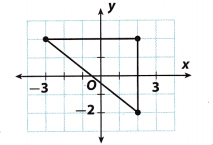Find the length of each leg. The length of the vertical leg is 4 units. The length of the horizontal Leg is 5 units. Let a = 4 and b = 5. Let c represent the length of the hypotenuse.
Use the Pythagorean Theorem to find c
a2 + b2 = c2 ……………. (1)
42 + 52 = c2 (Substitute into the formula) …………… (2)
16 + 25 = c2 (Simplify) …………. (3)
41 = c2 (Add) ………….. (4)
c = $$\sqrt {41}$$ (Take the square root of both sides) …………. (5)
c ≈ 6.4 (Use a calculator and round to the nearest tenth) ……………… (6)

Finding the Distance Between Any Two Points

The Pythagorean Theorem can be used to find the distance between any two points (x1, y1) and (x2, y2) in the coordinate plane. The resulting expression is called the Distance Formula.

Use the Pythagorean Theorem to derive the Distance Formula.
A. To find the distance between points P and Q, draw segment $$\overline{P Q}$$ and label its length d. Then draw horizontal segment $$\overline{P R}$$ and vertical segment $$\overline{Q R}$$. Label the lengths of these segments a and b. Triangle
PQR is a ______________ triangle, with hypotenuse ____________.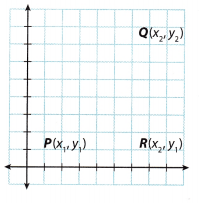B. Since $$\overline{P R}$$ is a horizontal segment, its length, a, is the difference between its x-coordinates. Therefore, a = x2 – ___________.
C. Since $$\overline{Q R}$$ is a vertical segment, its length, b, is the difference between its y-coordinates. Therefore, b = y2 – _____________.
D. Use the Pythagorean Theorem to find d, the length of segment $$\overline{P Q}$$. Substitute the expressions from B, and C for a and b.
d2 = a2 + b2
d = $$\sqrt{a^{2}+b^{2}}$$Reflect

Go Math Lesson 8.3 Distance Between Two Points Answer Key Question 2.
Why are the coordinates of point R the ordered pair (x2, y1)?
Since $$\overline{Q R}$$ is a vertical statement, the x coordinate of R will be the same as the x coordinate of Q. On the other hand, since $$\overline{P R}$$ is a horizontal statement, the y coordinate of R will be the same as the y coordinate of Q. Therefore, the coordinates of point R is the ordered pair (x2, y1).

Example 2

Francesca wants to find the distance between her house on one side of a lake and the beach on the other side. She marks off a third point forming a right triangle, as shown. The distances in the diagram are measured in meters.Use the Pythagorean Theorem to find the straight-line distance from Francesca’s house to the beach.
STEP 1: Find the length of the horizontal leg.
The length of the horizontal leg is the absolute value of the difference between the x-coordinates of the points (280, 20) and (10, 20).
|280 – 10| = 270
The length of the horizontal leg is 270 meters.

STEP 2: Find the length of the vertical leg.
The length of the vertical leg is the absolute value of the difference between they-coordinates of the points (280, 164) and (280, 20).
|164 – 20| = 144
The length of the vertical leg is 144 meters.

STEP 3: Let a = 270 and b = 144. Let c represent the length of the hypotenuse. Use the Pythagorean Theorem to find c.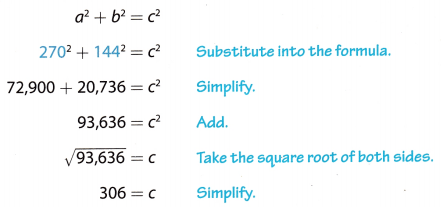The distance from Francesca’s house to the beach is 306 meters.

Reflect

Question 3.
Show how you could use the Distance Formula to find the distance from Francesca’s house to the beach.
You can use the Distance Formula, d = $$\sqrt{\left(x_{2}-x_{1}\right)^{2}+\left(y_{2}-y_{1}\right)^{2}}$$ , to find the distance between the house and the beach. Let (x1, y1) = (10, 20) and (x2, y2) = (280, 164).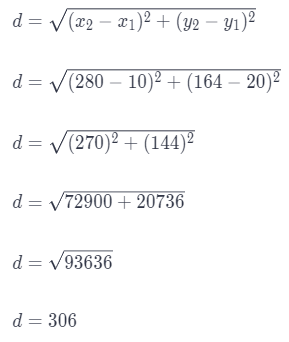Question 4.
Camp Sunshine is also on the lake. Use the Pythagorean Theorem to find the distance between Francesca’s house and Camp Sunshine to the nearest tenth of a meter.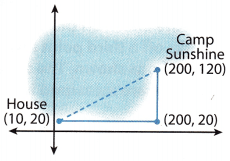Use the Pythagorean Theorem to find the straight-line distance from Francesca’s house to the Camp Sunshine. The length of the horizontal leg is the absolute value of the difference between the x-coordinates of the points (200, 20) and (10, 20).
|200 – 10| = 190
The length of the vertical leg is the absolute value of the difference between the y-coordinates of the points (200, 120) and (200, 20).
|120 – 20| = 100
Let = 190, b = 100, and Let c represent the Length of the hypotenuse. Use the Pythagorean Theorem to find c.
a2 + b2 = c2 …………….. (1)
1902 + 1002 = c2 (Substitute into the formula) …………. (2)
36100 + 10000 = c2 (Simplify) …………… (3)
46100 = c2 (Add) ………….. (4)
c = $$\sqrt {46100}$$ (Take the square root of both sides) ………… (5)
c ≈ 214.7 (Use a calculator and round to the nearest tenth) ……………. (6)
The distance from Francesca’s house and Camp Sunshine is approximately 214.7 meters.

Question 1.
Approximate the length of the hypotenuse of the right triangle to the nearest tenth without using a calculator. (Example 1).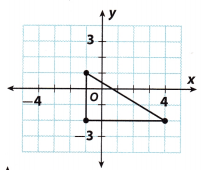The length of the vertical leg is 3 units. The length of the horizontal Leg is 5 units. Let a = 3 and b = 5. Let c represent the length of the hypotenuse. We use the Pythagorean Theorem to find c.
a2 + b2 = c2
32 + 52 = c2
9 + 25 = c2
34 = c2
c = $$\sqrt {34}$$
Approximate $$\sqrt {34}$$ by finding perfect squares close to 34.
25 < 34 < 36
$$\sqrt {25}$$ < $$\sqrt {34}$$ < $$\sqrt {36}$$
5 < $$\sqrt {34}$$ < 6
$$\sqrt {34}$$ ≈ 5.5
The hypotenuse is about 5.5 units long.

Go Math Lesson 8.3 8th Grade Distance between Two Points Answer Key Question 2.
Find the distance between the points (3, 7) and (15, 12) on the coordinate plane. (Explore Activity).
Using the Distance Formula we can find the distance between points (x1, y1) = (3, 7) and (x2, y2) = (15, 12) in the coordinate plane.The distance between the points is 13 units.

Question 3.
A plane leaves an airport and flies due north. Two minutes later, a second plane leaves the same airport flying due east. The flight plan shows the coordinates of the two planes 10 minutes later. The distances in the graph are measured in miles. Use the Pythagorean Theorem to find the distance shown between the two planes. (Example 2)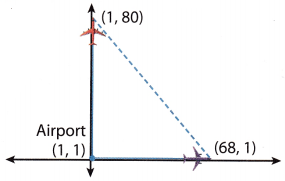Use the Pythagorean Theorem to find the straight-line distance between two planes.
The length of the vertical leg is distance between first plane and the starting point: the absolute value of the difference
between the y-coordinates of the points (1, 80) and (1, 1).
|80 – 1| = 79
The length of the horizontal leg is distance between second plane and the starting point: it is the absolute value of the difference between the x-coordinates of the points (68, 1) and (1, 1).
|68 – 1| = 67
Let a = 79, b = 67, and let c represent the Length of the hypotenuseUse the Pythagorean Theorem to find c.
a2 + b2 = c2 ……………….. (1)
792 + 672 = c2 (Substitute into the formula) ……………. (2)
6241 + 4489 = c2 (Simplify) ……………. (3)
10730 = c2 (Add) ………………. (4)
c = $$\sqrt {10730}$$ (Take the square root of both sides) …………….. (5)
c ≈ 103.6 (Use a calculator and round to the nearest tenth) ……………… (6)
The distance between two planes is approx 103.6 miles.

Essential Question Check-In

Question 4.
Describe two ways to find the distance between two points on a coordinate plane.
You can draw a right triangle whose hypotenuse is the segment connecting the two points and then use the Pythagorean Theorem to find the length of that segment. You can also use the Distance Formula to find the length of that segment.
For example, plot three points; (1, 2), (20, 2), and (20, 12)
Using the Pythagorean Theorem
The length of the horizontal leg is the absolute value of the difference between the x-coordinates of the points (1, 2) and (20, 2).
|1 – 20| = 19
The length of the horizontal leg is 10.
Let a = 19, b = 10 and Let c represent the hypotenuse. Find c.
a2 + b2 = c2
192 + 102 = c2
361 + 100 = c2
461 = c2
distance → 21.5 ≈ e
Using the Distance Formula
d = $$\sqrt{\left(x_{2}-x_{1}\right)^{2}+\left(y_{2}-y_{1}\right)^{2}}$$
The length of the horizontal leg in between (1, 2) and (20, 2).The length of the horizontal leg in between (20, 2) and (20, 12).The length of the horizontal leg in between (1, 2) and (20, 12).Question 5.
A metal worker traced a triangular piece of sheet metal on a coordinate plane, as shown. The units represent inches. What is the length of the longest side of the metal triangle? Approximate the length to the nearest tenth of an inch without using a calculator.(5 × 5) + (6 × 6) = c × c
25 + 36 = c × c
$$\sqrt {61}$$ = c
c ≈ 7.8

Go Math Lesson 8.3 Using Midpoint and Distance Formulas Answers Question 6.
When a coordinate grid is superimposed on a map of Harrisburg, the high school is located at (17, 21) and the town park is located at (28, 13). If each unit represents 1 mile, how many miles apart are the high school and the town park? Round your answer to the nearest tenth.
The coordinates of the high school, are said to be (17, 21), whereas the coordinates of the park are (28, 13). In a coordinate plane, the distance d between the points (17, 21) and (28, 13) is:
d = $$\sqrt{\left(x_{2}-x_{1}\right)^{2}+\left(y_{2}-y_{1}\right)^{2}}$$
d = $$\sqrt{(28-17)^{2}+(13-21)^{2}}$$
d = $$\sqrt{(11)^{2}+(-8)^{2}}$$
d = $$\sqrt{121+64}$$
d = $$\sqrt{185}$$
Rounding the answer to the nearest tenth:
d ≈ 13.6
Taking into consideration that each unit represents 1 mile, the high school and the town park are 13.6 miles apart.

Question 7.
The coordinates of the vertices of a rectangle are given by R(-3, -4), E(-3, 4), C(4, 4), and T(4, -4). Plot these points on the coordinate plane at the right and connect them to draw the rectangle. Then connect points E and T to form diagonal $$\overline{E T}$$.a. Use the Pythagorean Theorem to find the exact length of $$\overline{E T}$$.Taking into consideration the triangLe TRE, the Length of the vertical leg ($$\overline{E R}$$) is 8 units. The Length of the horizontal. Leg ($$\overline{R T}$$) is 7 units. Let a = 8 and b = 7. Let c represent the length of the hypotenuse, the diagonal
$$\overline{E T}$$. We use the Pythagorean Theorem to find c.
a2 + b2 = c2
82 + 72 = c2
64 + 49 = c2
113 = c2
c = $$\sqrt {113}$$
c ≈ 10.6
The diagonal $$\overline{E T}$$ is about 10.63 units long.

b. How can you use the Distance Formula to find the length of $$\overline{E T}$$? Show that the Distance Formula gives the same answer.
Using the distance Formula in a coordinate plane, the distance d between the points E(-3, 4) and T(4, 4)
d = $$\sqrt{\left(x_{2}-x_{1}\right)^{2}+\left(y_{2}-y_{1}\right)^{2}}$$
d = $$\sqrt{(4-(-3))^{2}+(-4-4)^{2}}$$
d = $$\sqrt{(7)^{2}+(-8)^{2}}$$
d = $$\sqrt{49+64}$$
d = $$\sqrt{113}$$
d ≈ 10.63
The diagonal $$\overline{E T}$$ is about 10.63 units long.
As we can see, the answer is the same as the one we found on point a.

Question 8.
Multistep The locations of three ships are represented on a coordinate grid by the following points: P(-2, 5), Q(-7, -5), and R(2, -3). Which ships are farthest apart?
Distance Formula: In a coordinate plane, the distance d between two points (x1, y1) and (x2, y1) is:
d = $$\sqrt{\left(x_{2}-x_{1}\right)^{2}+\left(y_{2}-y_{1}\right)^{2}}$$
The distance d1 between the points P(2, 5) and Q(-7, -5) is: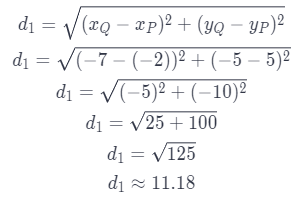The distance d2 between the points Q(-7, -5) and R(2, -3) is: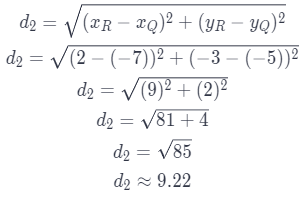The distance d3 between the points P(-2, 5) and R(2, -3) is: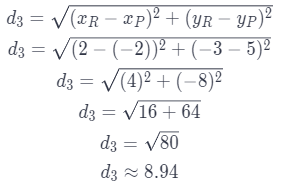As we can see, the greatest distance is d1 ≈ 11.8, which means that ships P and Q are farthest apart.

Go Math 8th Grade Distance and Midpoint Map Activity Answer Key Question 9.
Make a Conjecture Find as many points as you can that are 5 units from the origin. Make a conjecture about the shape formed if all the points 5 units from the origin were connected.
Some of the points that are 5 units away from the origin are: (0, 5), (3, 4), (4, 3), (5, 0), (4, 3), (3, -4), (0, 5) (-3, 4), (-4, 3), (- 5, 0), ( 4, 3), (-3, 4) etc. If all the points 5 units away from the origin are connected, a circle would be formed.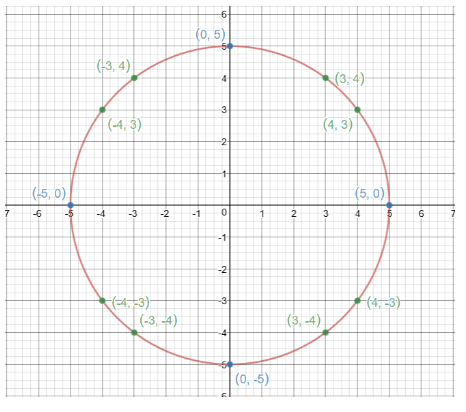Question 10.
Justify Reasoning The graph shows the location of a motion detector that has a maximum range of 34 feet. A peacock at point P displays its tail feathers. Will the motion detector sense this motion? Explain.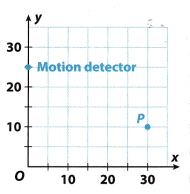The coordinates of the motion detector are said to be (0, 25), whereas the coordinates of the peacock are (30, 10). In a coordinate plane, the distance d between the points (0, 25) and (30, 10) is:
d = $$\sqrt{\left(x_{2}-x_{1}\right)^{2}+\left(y_{2}-y_{1}\right)^{2}}$$
d = $$\sqrt{(30-0)^{2}+(10-25)^{2}}$$
d = $$\sqrt{(30)^{2}+(-15)^{2}}$$
d = $$\sqrt{900+225}$$
d = $$\sqrt{1125}$$
Rounding the answer to the nearest tenth:
d ≈ 33.5
Taking into consideration that each unit represents 1 foot,
the motion detector and the peacock are 33.5 feet apart. Since the motion detector has a maximum range of 34 feet, it means that it wilt sense the motion of the peacock’s feathers.

H.O.T. Focus on Higher Order Thinking

Question 11.
Persevere in Problem-Solving One leg of an isosceles right triangle has endpoints (1, 1) and (6, 1). The other leg passes through the point (6, 2). Draw the triangle on the coordinate plane below. Then show how you can use the Distance Formula to find the length of the hypotenuse. Round your answer to the nearest tenth.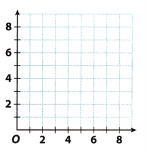One leg of an isosceles right triangle has endpoints (1, 1) and (6. 1), which means that that leg is 5 units long. Since the triangle is isosceles, the other leg should be 5 units long too, therefore the endpoints of the second leg that passes through point (6, 2) are (6, 1) and (6, 6).
In the coordinate plane, the length of the hypotenuse is the distance d between the points (1, 1) and (6, 6).
d = $$\sqrt{\left(x_{2}-x_{1}\right)^{2}+\left(y_{2}-y_{1}\right)^{2}}$$
d = $$\sqrt{(6-1)^{2}+(6-1)^{2}}$$
d = $$\sqrt{(5)^{2}+(5)^{2}}$$
d = $$\sqrt{25+25}$$
d = $$\sqrt{50}$$
Rounding the answer to the nearest tenth:
d ≈ 7.1
The hypotenuse is around 7.1 units long.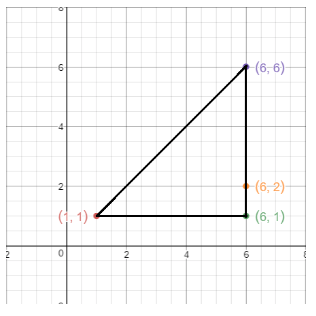Question 12.
Represent Real-World Problems The figure shows a representation of a football field. The units represent yards. A sports analyst marks the locations from where the football was thrown (point A) and where it was caught (point B). Explain how you can use the Pythagorean Theorem to find the distance the ball was thrown. Then find the distance.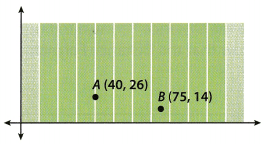To find the distance between points A and B, we draw segment $$\overline{A B}$$ and label its length d. Then we draw vertical segment $$\overline{A C}$$ and horizontal segment $$\overline{C B}$$. We label the lengths of these segments a and b. Triangle ACB is a right triangle with hypotenuse AB.
Since $$\overline{A C}$$ is a vertical segment, its length, a, is the difference between its y-coordinates. Therefore, a = 26 – 14 = 12 units.
Since $$\overline{C B}$$ is a horizontal segment, its length, b, is the difference between its x-coordinates. Therefore, b = 75 – 40 = 35 units.
We use the Pythagorean Theorem to find d, the length of segment $$\overline{A B}$$.
d2 = a2 + b2
d2 = 122 + 352
d2 = 144+ 1225
d2 = 1369
d = $$\sqrt {1369}$$
d = 37
The distance between points A and B is 37 yards.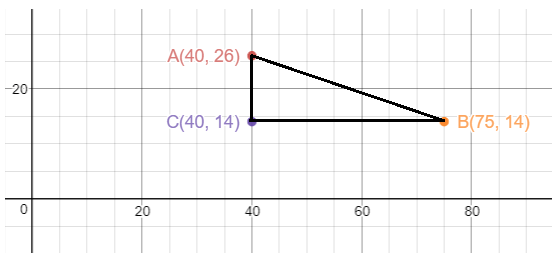Scroll to Top